# Using the MortalityTables Package

#### 2023-10-27

required <- c("tidyverse")
if (!all(sapply(required,
function(pkg) requireNamespace(pkg, quietly = TRUE)))) {
message(paste("This vignette needs the followig packages:\n\t",
paste(required, collapse = " "),
"\nSince not all are installed, code will not be executed: "))
knitr::opts_chunk$set(eval = FALSE) } The MortalityTables package provides the mortalityTable base class and some derived classes to handle different types of mortality tables (also called life tables), mainly used for life insurance. Additionally it provides a plot function to compare multiple life tables either directly using the absolute mortalities in log-linear plots or using relative mortalities as percentages of a given reference table. # 1 Types of Life Tables Provided types of mortality tables are: • Base class Class mortalityTable • Period life table Class mortalityTable.period(ages, deathProbs, ..., baseYear=2000) Death probabilities observed / predicted for one observation year; No dependency on the bith year is assumed. • Cohort life table using age-specific trends Class mortalityTable.trendProjection Death probabilities of a given base year are projected into the future using age-specific trends $$\lambda_x$$. The death probability of an $$x$$-year old in year baseYear + n is calculated as: $q_x^{(baseYear+n)} = q_x^{(baseYear)} \cdot e^{-n\cdot\lambda_x}$ Consequently, the death probabilities for a person born in year YOB can be calculated as $q_x^{YOB} = q_x^{(base)} \cdot e^{-(YOB+x-baseYear)\cdot \lambda_x}$ • Cohort life table approximation using age shift Class mortalityTable.ageShift Death probabilities for cohort $$YOB$$ are obtained by using death probabilities for cohort $$X$$ and modifying the technical age with a birth-year dependent shift: $q_x^{YOB} = q_{x+shift(YOB)}^{(base)}$ • Observed life table Class mortalityTable.observed Death probabilities observed during several years. The probabilities are stored as a matrix with observation year and age as dimensions. • Mixed life table Class mortalityTable.mixed Arithmetic mean of two life tables with given weights. This approach is often used to generate unisex life tables by mixing male and female mortalities with given weights (e.g. 70:30 or 40:60) • Cohort life table using age-specific improvement factors Class mortalityTable.improvementFactors Project base life table using age-specific improvement factors. • Pension tables Class pensionTable Transition probabilities for a four-state pension model (active, invalid, retirement and death, with a possible widow in the event of death). # 2 Loading the MortalityTables package library("MortalityTables") # 3 Provided Data Sets The package provides several real-life life tables published by census bureaus and actuarial associations around the world. You can use the function mortalityTables.list to list all available datasets (if no argument is given) or all datasets that match the given pattern (wildcard character is *). You can then use mortalityTables.load to load either one single data set or all datasets that match the pattern. # list all available data sets mortalityTables.list() #>  "Austria_Annuities" "Austria_Annuities_AVOe1996R" #>  "Austria_Annuities_AVOe2005R" "Austria_Annuities_EROMF" #>  "Austria_Annuities_RR67" "Austria_Census" #>  "Austria_Endowments_ADSt2426_2Lives" "Austria_PopulationForecast" #>  "Austria_PopulationMCMC" "Austria_PopulationObserved" #>  "Austria_VUGesamtbestand_2012-16" "Germany_Annuities" #>  "Germany_Annuities_DAV1994R" "Germany_Annuities_DAV2004R" #>  "Germany_Census" "Germany_Endowments" #>  "Germany_Endowments_DAV1994T" "Germany_Endowments_DAV2008T" #>  "USA_Annuities" "USA_Annuities_1971IAM" #>  "USA_Annuities_1983a" "USA_Annuities_1994GAR" #>  "USA_Annuities_2012IAM" "USA_Annuities_Annuity2000" # list all datasets for Austria mortalityTables.list("Austria_*") #>  "Austria_Annuities" "Austria_Annuities_AVOe1996R" #>  "Austria_Annuities_AVOe2005R" "Austria_Annuities_EROMF" #>  "Austria_Annuities_RR67" "Austria_Census" #>  "Austria_Endowments_ADSt2426_2Lives" "Austria_PopulationForecast" #>  "Austria_PopulationMCMC" "Austria_PopulationObserved" #>  "Austria_VUGesamtbestand_2012-16" # Load the German annuity table DAV 2004-R mortalityTables.load("Germany_Annuities_DAV2004R") # Load all Austrian annuity data sets mortalityTables.load("Austria_Annuities*") mortalityTables.load("Austria_Census") In the next few sections we will always use some of the provided life tables for demonstration purposes. # 4 Working with life table objects ## 4.1 Plotting life tables The package provides several functions to plot lifetables: • plotMortalityTables(table1, table2, ...) A log-linear plot comparing all given life tables. • plotMortalityTableComparisons(table1, table2, ..., reference=reftable) Plot the given life tables as percentages relative to the reference table • plotMortalityTrend(table1, table2, ..., YOB, Period) Plot the yearly mortality improvement factors (for either the given observation year Period or the birth-year YOB) These functionalities are also combined into the S3 plot function for the mortalityTable class, so you can usually just call plot on the mortality tables. If the trend = TRUE argument is given, plotMortalityTrend is used, if the reference argument is given, plotMortalityTableComparisons is used, otherwise plotMortalityTables is called. # Log-linear plot comparing some Austrian census tables plot(mort.AT.census.1951.male, mort.AT.census.1991.male, mort.AT.census.2001.male, mort.AT.census.2011.male, legend.position = c(1,0))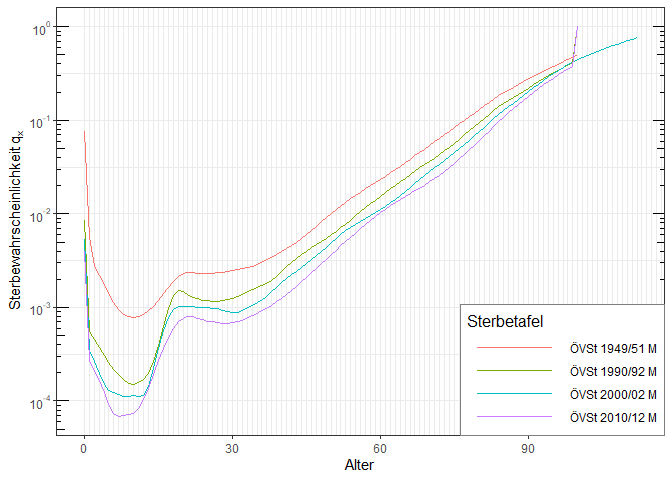# Relative death probabilities in percentage of the latest census plot(mort.AT.census.1951.male, mort.AT.census.1991.male, mort.AT.census.2001.male, reference = mort.AT.census.2011.male, legend.position = c(1,0.75), ylim = c(0,4)) #> Warning in normalize_deathProbabilities(if (is.data.frame(t@data$dim) || :
#> Reference mortality table does not contain ages
#> 101102103104105106107108109110111112 required for normalization. These ages
#> will not be normalized!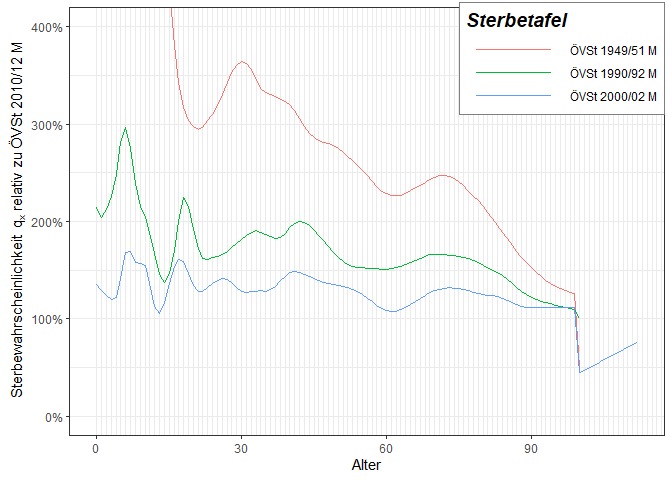For cohort life tables, the plot functions also take either the YOB or the Period parameter to plot either the cohort death probabilities for the given birth year or the period death probabilities for the given observation year.

# Comparison of two Austrian annuity tables for birth year 1977
plot(AVOe1996R.male, AVOe2005R.male, YOB = 1977, title = "Comparison for YOB=1977")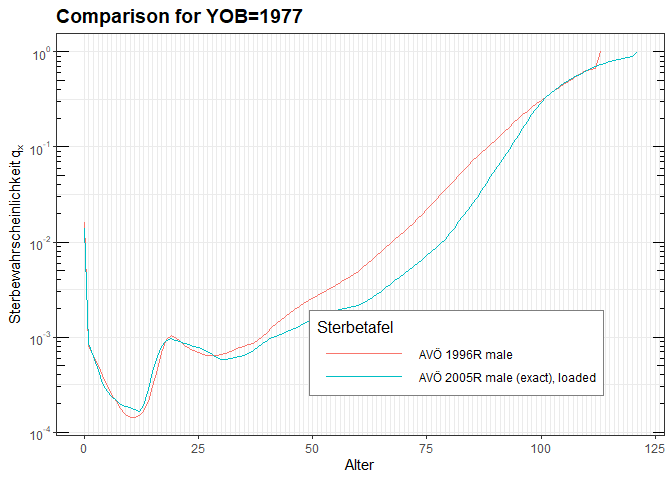# Comparison of two Austrian annuity tables for observation year 2020
plot(AVOe1996R.male, AVOe2005R.male, Period = 2020, title = "Comparison for observation year 2020")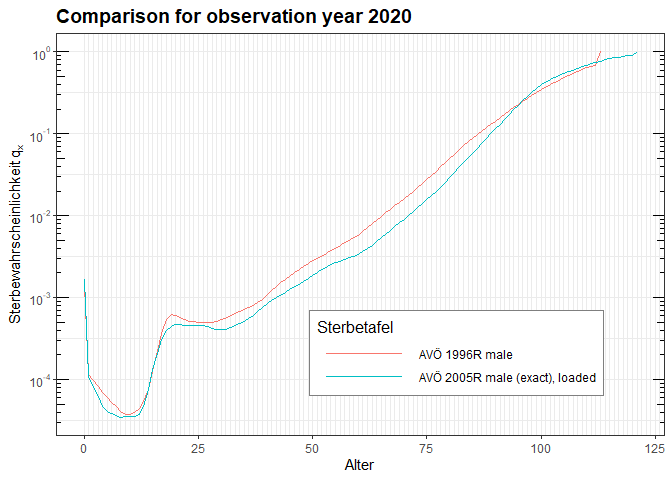## 4.2 Obtaining period and cohort death probabilities

To obtain death probabilities from all the different types of tables, there are two functions:

• deathProbabilities: Returns the (cohort) death probabilities of the life table given the birth year
• periodDeathProbabilities: Returns the (period) death probabilities of the life table for a given observation year
mortalityTables.load("Austria_Annuities")
# Get the cohort death probabilities for Austrian Annuitants born in 1977:
qx.coh1977 = deathProbabilities(AVOe2005R.male, YOB = 1977)

# Get the period death probabilities for Austrian Annuitants observed in the year 2020:
qx.per2020 = periodDeathProbabilities(AVOe2005R.male, Period = 2020)

These functions return the death probabilities as a simple, numeric R vector.

There are two similar functions that return the death probabilities as a period life table object that can be used with all other functions provided by this package:

• getCohortTable: Get a mortalityTable object describing the death probabilities for people born in the given year
• getPeriodTable: Get a mortalityTable object describing the death probabilities observed in the given year
# Get the cohort death probabilities for Austrian Annuitants born in 1977 as a mortalityTable.period object:
table.coh1977 = getCohortTable(AVOe2005R.male, YOB = 1977)

# Get the period death probabilities for Austrian Annuitants observed in the year 2020:
table.per2020 = getPeriodTable(AVOe2005R.male, Period = 2020)

# Compare those two in a plot:
plot(table.coh1977, table.per2020, title = "Comparison of cohort 1977 with Period 2020", legend.position = c(1,0))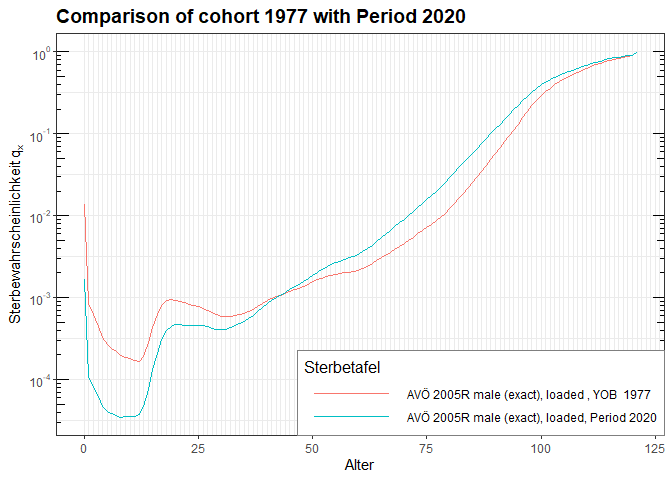Not surprisingly, at 43 years the two death probabilities cross, because in 2020 the person born 1977 is 43 years old, so the $$q_x$$ refer to the same person. However, for younger ages, the period 2020 probabilities are lower, because the mortality improvement for those younger ages has much less time in the cohort 1977 table. For ages above 43 the cohort table describes the mortality further into the future than 2020, so there is more improvement and thus lower death probabilities for the cohort life table.

## 4.3 Other data extraction functions from life tables

function description
ages(table) Returns the vector of ages, for which the life table can provide death probabilities
getOmega(table) Returns the maximum age, for which the life table can provide dath probabilities
ageShift(table, YOB) Returns the age shift for the given year of birth
baseTable(table) Returns the base table, from which the table projects (for cohort tables)
baseYear(table) Returns the year of the base table
lifetable(table, YOB) Returns the cohort death probabilities as a lifetable object for use with the lifecontingencies package

## 4.4 Dimensional information

Mortality tables are always created for special purposes, particular collectives, types of risk, sex, year, etc. So, each MortalityTable object provides for a list of such factors that describe the underlying target of the mortality table and that can be used e.g. when plotting mortality Tables (just like any other factor variable in a ggplot):

plotMortalityTables(
mort.AT.census[c("m", "w"), c("1951", "1991", "2001", "2011")]) +
aes(color = as.factor(year), linetype = sex) + labs(color = "Period", linetype = "Sex")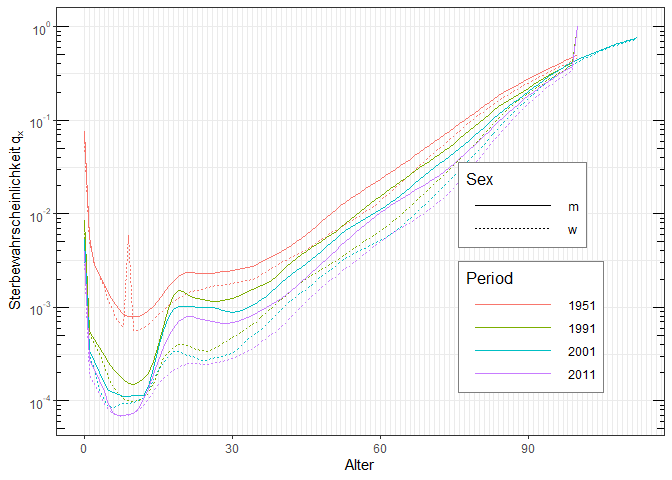The dimensional information is stored inside the @data$dim field of the MortalityTable: mort.AT.census.2011.male@data$dim
#> $sex #>  "m" #> #>$collar
#>  "Gesamtbevölkerung"
#>
#> $type #>  "Volkssterbetafel Österreich" #> #>$data
#>  "official"
#>
#> $year #>  2011 #> #>$table
#>  "ÖVSt 2010/12"

There are no hard and enforced rules for these names and the potential values of the dimensional information. There are, however, some conventions that are obeyed by most of the tables provided by this package:

Key Potential values Description
sex “m”, “w”, “u” Sex
collar “Rententafel”, “Gruppenrententafel”, “Einzel”, “Gruppe”, “Gesamtbevölkerung”, “Raucher”, “Nichtraucher”, “Arbeiter”, “Angestellte”, “Mischtafel” Collective, to which the mortality table applies
type “Rententafel”, “Volkssterbetafel”, “Pensionstafel”, “Bevölkerungsprognose”, “Beobachtung”, “Risikotafel” The type of table
data “official”, “raw”, “loaded”, “loaded, group”, “unloaded”, “age-shifted”, “geglättet” The type of data
year numeric year, “2014-2080”, “1980-2017”, “1947-2017” The year (or range) described by the table
tablename “AVÖ 1996-R”, “AVÖ 2005-R”, “EROM 85”, “EROF 85”, “EROM G1950”, “EROF G1950”, “EROM G1950 AV”, “EROF G1950 AV”, “RR67”, “DAV 1994R”, “DAV 2004R”, “DAV 1994T”, “DAV 2008T”, “1971 IAM”, “1971 IAM projected”, “1983a”, “1983 GAM”, “1994 GAM”, “1994 GAR”, “2012 IAM”, “Annuity 2000”, “AVÖ 1999-P”, “AVÖ 2008-P”, “Ettl-Pagler 1989”, “DAV 2005-G” The formal name of the table
risk “Tod”, “sonst. Ausscheiden”, “Invalidisierung”, “Partnerwahrscheinlichkeit im Tod”, “mittl. Hinterbliebenenalter” The type of risk described by the table
probability “qx”, “sx”, “ix”, “qgx”, “qix”, “qpx”, “hx”, “qwy”, “yx” The probability described by the table (corresponds with “risk”)
country “Österreich”, “Deutschland”, “USA”, … The geographic region of the table (not neccessarily only countries)
source “AVÖ”, “Statistik Austria”, “DAV”, … Source of the data / table

Some of the provided datasets (mortality tables) have not yet fully implemented these conventions, so pleasy be vary when using them.

# 5 Creating a life table object

## 5.1 Period life tables

Period death probabilities are the simplest type of life table, giving the probabilities of death observed during the corresponding year (the “period”). The death probabilities of different ages refer to different persons, being of the corresponding ages in the observation year. All that is needed to create a period life table are the death probabilities and the corresponding ages:

lt = mortalityTable.period(name = "Sample period lifetable", ages = 1:99, deathProbs = exp(-(99:1)/10))
plot(lt, title = "Simple log-linear period mortality table")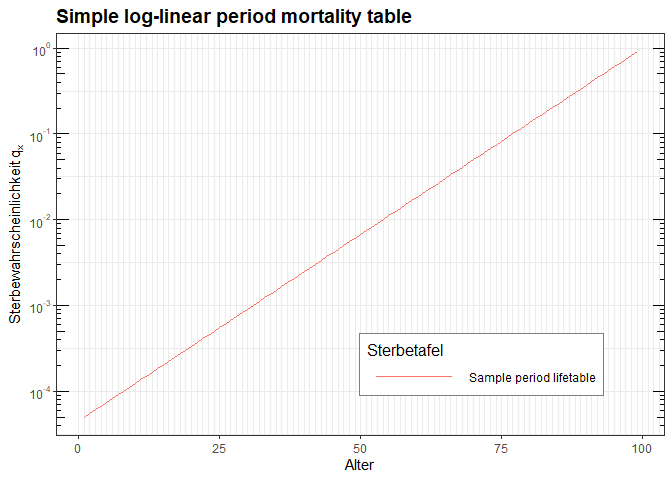deathProbabilities(lt)
#>   5.017468e-05 5.545160e-05 6.128350e-05 6.772874e-05 7.485183e-05
#>   8.272407e-05 9.142423e-05 1.010394e-04 1.116658e-04 1.234098e-04
#>  1.363889e-04 1.507331e-04 1.665858e-04 1.841058e-04 2.034684e-04
#>  2.248673e-04 2.485168e-04 2.746536e-04 3.035391e-04 3.354626e-04
#>  3.707435e-04 4.097350e-04 4.528272e-04 5.004514e-04 5.530844e-04
#>  6.112528e-04 6.755388e-04 7.465858e-04 8.251049e-04 9.118820e-04
#>  1.007785e-03 1.113775e-03 1.230912e-03 1.360368e-03 1.503439e-03
#>  1.661557e-03 1.836305e-03 2.029431e-03 2.242868e-03 2.478752e-03
#>  2.739445e-03 3.027555e-03 3.345965e-03 3.697864e-03 4.086771e-03
#>  4.516581e-03 4.991594e-03 5.516564e-03 6.096747e-03 6.737947e-03
#>  7.446583e-03 8.229747e-03 9.095277e-03 1.005184e-02 1.110900e-02
#>  1.227734e-02 1.356856e-02 1.499558e-02 1.657268e-02 1.831564e-02
#>  2.024191e-02 2.237077e-02 2.472353e-02 2.732372e-02 3.019738e-02
#>  3.337327e-02 3.688317e-02 4.076220e-02 4.504920e-02 4.978707e-02
#>  5.502322e-02 6.081006e-02 6.720551e-02 7.427358e-02 8.208500e-02
#>  9.071795e-02 1.002588e-01 1.108032e-01 1.224564e-01 1.353353e-01
#>  1.495686e-01 1.652989e-01 1.826835e-01 2.018965e-01 2.231302e-01
#>  2.465970e-01 2.725318e-01 3.011942e-01 3.328711e-01 3.678794e-01
#>  4.065697e-01 4.493290e-01 4.965853e-01 5.488116e-01 6.065307e-01
#>  6.703200e-01 7.408182e-01 8.187308e-01 9.048374e-01

## 5.2 Cohort life tables with trend projection

A cohort life table with trend projection needs the following parameters:

• The base table $$q_x^{(base)}$$ (death probabilities) for the given base period as a vector
• Age-specific trend factors $$\lambda_x$$ as a vector
• The base year (numeric)
atPlus2 = mortalityTable.trendProjection(
name = "Austrian Census Males 2011, 2% yearly trend",
baseYear = 2011,
deathProbs = deathProbabilities(mort.AT.census.2011.male),
ages = ages(mort.AT.census.2011.male),
trend = rep(0.02, length(ages(mort.AT.census.2011.male)))
)

Some life tables do not assume a constant age-specific trend over time, but rather assume that the currently observed high mortality improvements are just a temporary effect, so the current trend is in effect only for some time and then reduces to some kind of long-term trend.

There are two conceptual approaches: One is to use a trend dampening function that is simply applied to the starting trend. So, while the initial trend might be 3%, i.e. the projection will use (ObservationYear-BaseYear) * OriginalYear, over time it will assume the value dampeningFunction(ObservationYear-BaseYear) * OriginalTrend. The dampening function in this case gives the cumulated trend effect from the base year until the observation year. To implement this trend reduction with the MortalityTables package, simply pass a one-argument function as the dampingFunction slot to the class, the argument will be the number of years from the base year (NOT the calendar year!):

atPlus2.damp = mortalityTable.trendProjection(
name = "Austrian M '11, 2% yearly, damping until 2111",
baseYear = 2011,
deathProbs = deathProbabilities(mort.AT.census.2011.male),
ages = ages(mort.AT.census.2011.male),
trend = rep(0.02, length(ages(mort.AT.census.2011.male))),
# damping function: 2011: full effect, linear reduction until yearly trend=0 in 2111:
# 2011: 100%, 2012: 99%, 2013: 98% => For 2013 we have a cumulative trend
# of 297% instead of 300% for three full yearly trends!
dampingFunction = function(n) { n - n * (n + 1) / 2 / 100 }
)

plot(mort.AT.census.2011.male, atPlus2, atPlus2.damp, YOB = 2011, legend.position = c(0.8,0.75))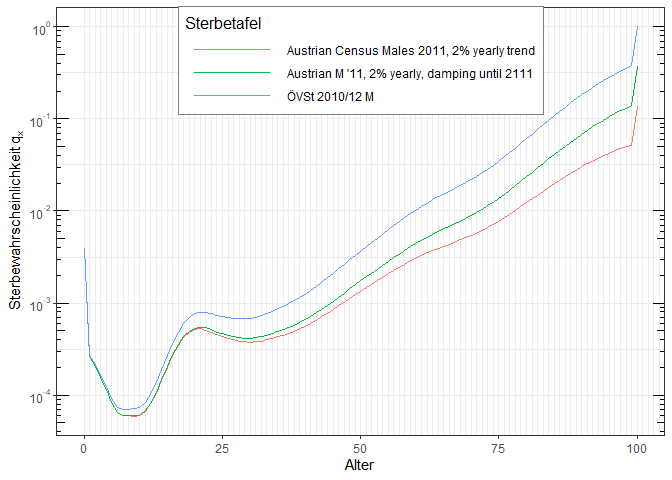The other approach is to assume that instead of the initial trend, after some time a second trend (slot trend2) takes over. In this case, the dampingFunction slot is again a one-argument function that now gives the weight of the first trend, while 1-dampingFunction(year) will give the weight of the second trend. As the weights will be applied for the whole period from the base- to the observation year, the weights need to be cumulated and normalized.

The argument in this case is the actual calendar year (not the year since the base year like it was in the one-trend case above!)

atPlus2.damp2 = mortalityTable.trendProjection(
name = "Austrian M '11, 2% yearly, 1% long-term",
baseYear = 2011,
deathProbs = deathProbabilities(mort.AT.census.2011.male),
ages = ages(mort.AT.census.2011.male),
trend = rep(0.02, length(ages(mort.AT.census.2011.male))),
trend2 = rep(0.01, length(ages(mort.AT.census.2011.male))),
# damping function interpolates between the two trends:
# until 2021 trend 1, from 2031 trend 2, linearly beteen
dampingFunction = function(year) {
if (year <= 2021) 1
else if (year > 2031) 14.5/(year - 2011)
else 1 - (year - 2021)*(year - 2021 + 1) / 20 / (year - 2011)
}
)

plot(mort.AT.census.2011.male, atPlus2, atPlus2.damp, atPlus2.damp2, YOB = 2011, legend.position = c(0.02, 0.98), legend.justification = c(0, 1))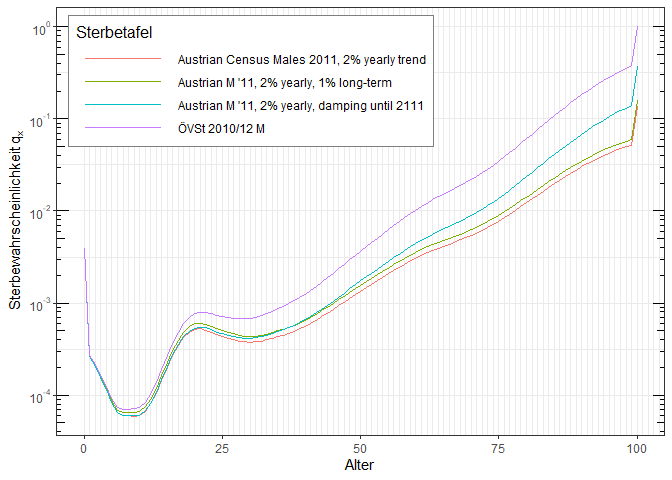## 5.3 Cohort life tables with age-shift

Age-shifted cohort life tables are an approximation to full cohort life tables. Full cohort life tables apply a trend or improvment factors to the death probabilities of a base year to obtail death probabilities for a given birth year. Age-shifting rather modifies the age of the corresponding person and uses the same, unmodified base table for all cohorts. Basically, it works like this:

A 60-year old born in 1950 has the same death probability as a 50-year old born in 1900, so instead of looking at the cohort 1950, we can look at the cohort 1900 and for a person born 1950 we treat him as if he were 10 years younger.

So, an age-shifted cohort life table just needs the base table and for each birth year the amount the age is modified.

For those people, who think visually, age shifting works on the death probabilities as following: A normal trend moves the $$q_x$$ curve downwards. Age-shifting approximates this by shifting the $$q_x$$ curve to the right without modifying its values.

The following example clearly shows this, with the blue curve being the base table for YOB 2011. A full trend projection moves the curve down to the green line, while age-shifting moves the base curve to the right so that it coincides as much as possible with the exact (green) line.

baseTableShift = getCohortTable(atPlus2, YOB = 2011);
baseTableShift@name = "Base table of the shift (YOB 2011)"

atShifted = mortalityTable.ageShift(
name = "Approximation with age shift",
baseYear = 2011,
deathProbs = deathProbabilities(baseTableShift),
ages = ages(baseTableShift),
ageShifts = data.frame(
shifts = c(
rep( 0, 3),
rep(-1, 3),
rep(-2, 3),
rep(-3, 3),
rep(-4, 3),
rep(-5, 3),
rep(-6, 3)
),
row.names = 2011:2031
)
)

ageShift(atShifted, YOB = 2021)
#>  -3

plot(baseTableShift, atPlus2, atShifted, YOB = 2021, legend.position = c(0.8,0.75))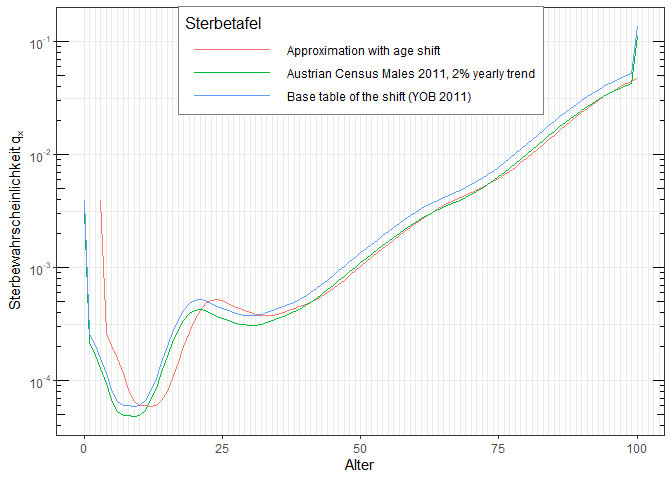As one can see, for ages above 40 years, the table with 2% yearly trend and the corresponding age-shifted table have roughly the same mortalities. Below 40 years, the two are very different, so this approximation through age-shifting should really be used with extreme care!

# 6 Modifying life table objects

## 6.1 Copying life tables

Life tables are simple pass-by-value S4 objects, so copying works by simple assignment.

b = AVOe2005R.female
b@name = "Modified Copy"
# only b is modified, not the original table
b@modification = function(qx) pmax(qx, 0.01)
plot(AVOe2005R.female, b, YOB = 2000)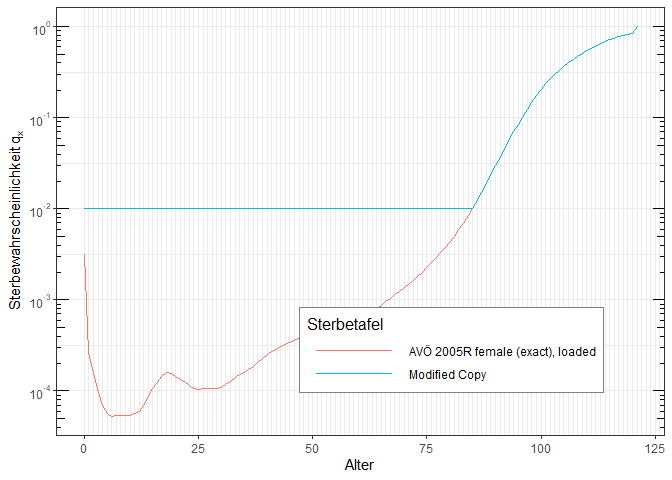When calculating premiums for life insurance contracts, one often needs to add a certain security loading on the raw death probabilities (e.g. 10% increased death probabilities) to account for statistical fluctuations. This can be easily done with the setLoading function that returns a copy of the given table and adds the given security loading.

AVOe2005R.female.sec = setLoading(AVOe2005R.female, loading = 0.1);
# Make sure the modified table has a new name, otherwise plots might break
plot(AVOe2005R.female, AVOe2005R.female.sec, title = "Original and modified table")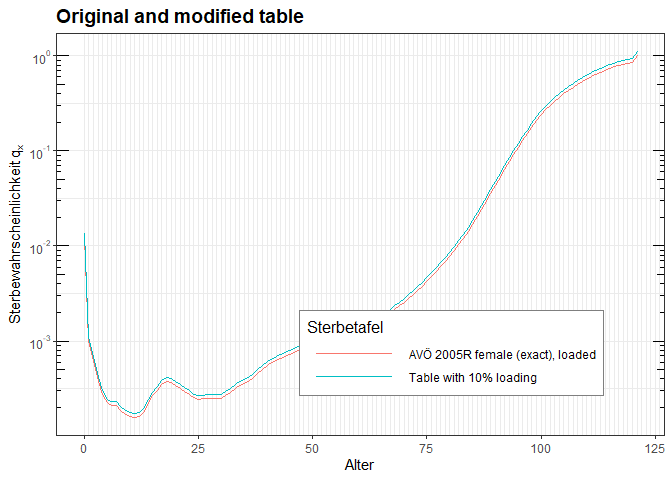## 6.3 Adding a modification to the raw probabilities

Some uses require post-processing of the death probabilities, like adding a lower bound for the death probabilities. To achive this, all mortalityTable-derived classes have a slot modification that takes a function that is passed the vector of death probabilities.

AVOe2005R.female.mod = setModification(AVOe2005R.female, modification = function(qx) pmax(0.03, qx));
# Make sure the modified table has a new name, otherwise plots might break
AVOe2005R.female.mod@name = "Modified table (lower bound of 3%)"
plot(AVOe2005R.female, AVOe2005R.female.mod, title = "Original and modified table")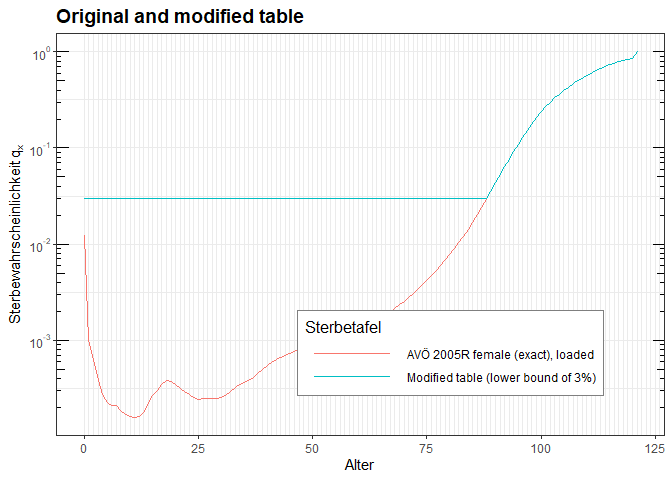# 7 Creating mortality tables from data and modifying them using various helper functions

The package MortalityTables not only provides the data structures and some examples of mortality tables, it also provides several functions to create mortality tables from raw data and modify them. The package provides several editing functions, which all begin with the prefix mT..

Let us take as an example the provided dataset PopulationData.AT2017 of Austrian population data (exposure and deaths counts for the year 2017).

For simplicity, we only look at the unisex data (i.e. male + female numbers, which are already provided as total exposure and total deaths). The raw mortality can then be calculated as

library(tidyverse)
data("PopulationData.AT2017", package = "MortalityTables")
PopulationData.AT2017.raw = PopulationData.AT2017 %>%
select(age, exposure.total, deaths.total) %>%
mutate(qraw = deaths.total / (exposure.total + deaths.total/2))

We now have all data needed to put it into a MortalityTable object (some fields like the exposre and the data list are not strictly needed, but can be useful later on):

PopulationTable.AT2017 = mortalityTable.period(
name = "Austrian Population Mortality 2017 (raw)",
baseYear = 2017,
deathProbs = PopulationData.AT2017.raw$qraw, ages = PopulationData.AT2017.raw$age,
exposures = PopulationData.AT2017.raw$exposure.total, data = list( deaths = PopulationData.AT2017.raw$deaths.total,
dim = list(sex = "u", collar = "Population", type = "raw", year = "2017")
)
)
plotMortalityTables(PopulationTable.AT2017, title = "Austrian population mortality (raw), 2017")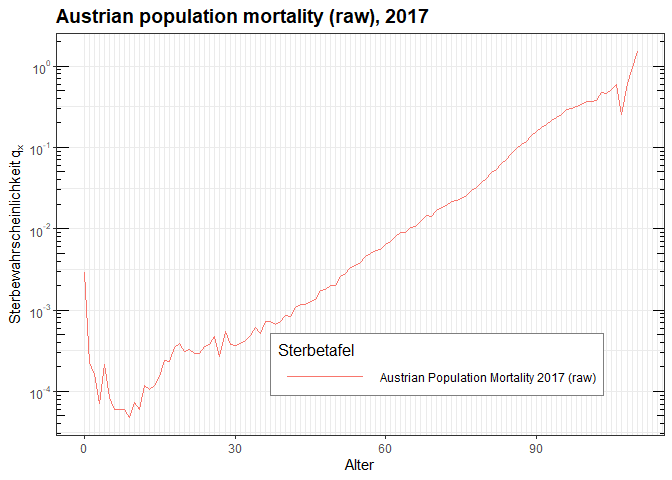Of course, we sooner or later want to work with a smooth table rather than the raw death probabilities. The most common approach to smoothing mortality tables is the Whittaker-Henderson method of graduation, which is provided by the function whittaker.mortalityTable(). The parameters are the $$\lambda$$ smoothing parameter (determining how smooth the result shall be, which in turn means that the result might be quite distant from the raw probabilities in some ages) and the order of differences $$d$$ (the default 2 typically suffices). Since we have the exposures available and stored inside the table, the whittaker.mortalityTable() function will use the exposures as weight and so try to match age ranges with high exposure much better than e.g. old ages with hardly any living.

PopulationTable.AT2017.smooth = PopulationTable.AT2017 %>%
whittaker.mortalityTable(lambda = 1/10, d = 2, name.postfix = ", Whittaker") %>%
mT.setDimInfo(type = "smoothed")
plotMortalityTables(PopulationTable.AT2017, PopulationTable.AT2017.smooth, title = "Austrian population mortality (raw and smoothed), 2017")  +
aes(colour = type)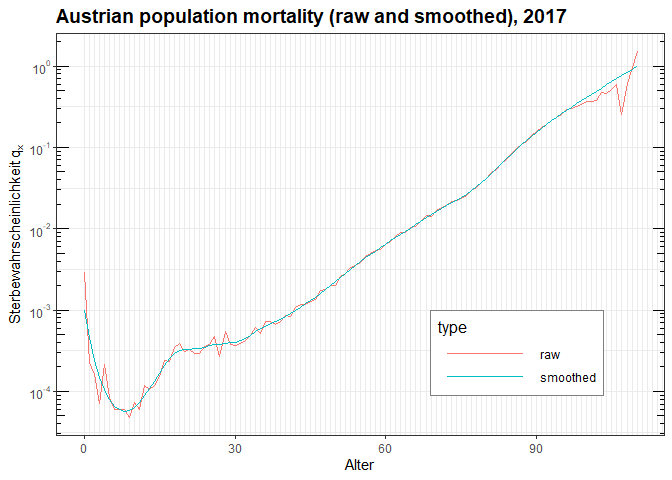As a side note, this example also shows how the additional dimensional infos set be either the constructor of the table or the mT.setDimInfo() function and stored in the table$data$dim list can be used by ggplot as aesthetics.

Now, if we look at the exposures, we see that above age 95 we are below an exposure of 5000 and at age 100 we are below exposure 500. So, for these old ages we apparently do not have enough data to derive mortalities with sufficient significance. So, let’s cut the table at age 100:

PopulationData.AT2017.raw %>% filter(age > 90)
#> # A tibble: 20 × 4
#>      age exposure.total deaths.total  qraw
#>    <dbl>          <dbl>        <dbl> <dbl>
#>  1    91       15616.           2963 0.173
#>  2    92       12870.           2721 0.191
#>  3    93       10245.           2451 0.214
#>  4    94        8024.           2113 0.233
#>  5    95        5875.           1727 0.256
#>  6    96        4041.           1382 0.292
#>  7    97        2480.            885 0.303
#>  8    98        1139.            433 0.320
#>  9    99         591.            237 0.334
#> 10   100         405.            182 0.367
#> 11   101         259.            116 0.366
#> 12   102         208.             98 0.382
#> 13   103         132.             82 0.473
#> 14   104          70.8            42 0.458
#> 15   105          37.7            26 0.513
#> 16   106          16.9            14 0.586
#> 17   107           6.98            2 0.251
#> 18   108           3.37            3 0.616
#> 19   109           0.8             0 0
#> 20   110           0.17            1 1.49
PopulationTable.AT2017.cut = PopulationTable.AT2017.smooth %>%
mT.fillAges(0:99) %>%
mT.setName("Austrian Population Mortality 2017, Whittaker-smoothed and cut at age 99")

Even though we don’t have enough statistical data to derive significant mortalities above 100, we still want to create a table that covers this age range by extrapolating the significant table to higher ages. This is typically done by selecting a fitting function and an appropriate age range, where the function is fit to the data. The parameters of the function calibrated to match the mortalities in the fitting range as good as possible are then used to extrapolate the mortalities with the function to ages outside the existing table.

The function mT.fitExtrapolationLaw uses the package MortalityLaws and the function MortalityLaws::MortalityLaw() to fit one of the mortality laws (see MortalityLaws::availableLaws() for all available laws) to the data and use that law to extrapolate to the desired ages, with a potential feding-in or fading-out age range.

In this example, we fit a Heligman-Pollard-type law (HP2) to the raw data and use it to extrapolate up to age 120. The age rante 80–95 is used to linearly switch from the (smoothed) death probabilities of the input table to the death probabilities calculated from the fitted law. So in this case, all observed probabilities above age 95 are not used at all anyway.

PopulationTable.AT2017.ex = PopulationTable.AT2017.smooth %>%
mT.fitExtrapolationLaw(law = "HP2", fit = 75:99, extrapolate = 80:120, fadeIn = 80:95) %>%
mT.setDimInfo(type = "smoothed and extrapolated")
plotMortalityTables(PopulationTable.AT2017, PopulationTable.AT2017.smooth, PopulationTable.AT2017.ex, title = "Austrian population mortality (raw and smoothed), 2017")  +
aes(colour = type)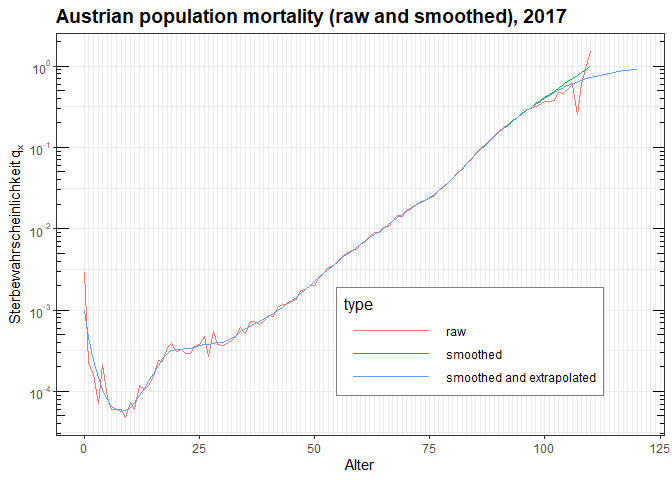Using different laws and different fitting age ranges can result in quite different results, so be carefull when extrapolating the table and always do a sanity-check on the results!

plotMortalityTables(
PopulationTable.AT2017,
PopulationTable.AT2017.smooth %>%
mT.fitExtrapolationLaw(law = "HP2", fit = 75:99, extrapolate = 80:120, fadeIn = 80:95) %>%
mT.setDimInfo(type = "Extrapolation: HP2, Fit 75--99"),

PopulationTable.AT2017.smooth %>%
mT.fitExtrapolationLaw(law = "HP2", fit = 75:85, extrapolate = 80:120, fadeIn = 80:95) %>%
mT.setDimInfo(type = "Extrapolation: HP, Fit 75--85"),

PopulationTable.AT2017.smooth %>%
mT.fitExtrapolationLaw(law = "HP2", fit = 90:110, extrapolate = 80:120, fadeIn = 90:100) %>%
mT.setDimInfo(type = "Extrapolation: HP2, Fit 90--110"),

title = "Examples of different fitting ranges for extrapolation")  +
aes(colour = type)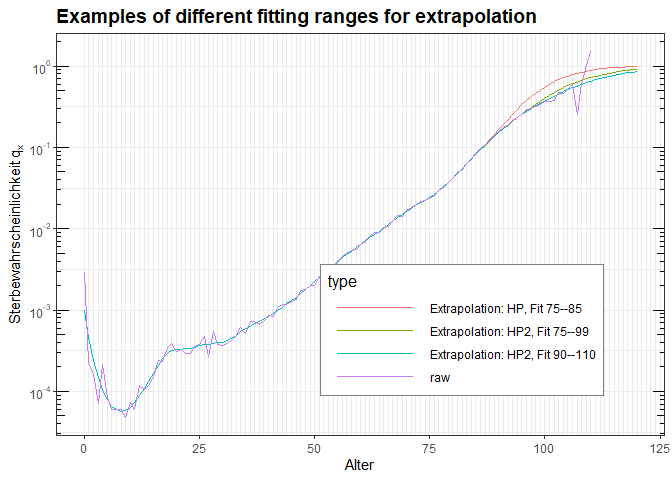plotMortalityTables(
PopulationTable.AT2017,
PopulationTable.AT2017.smooth %>%
mT.fitExtrapolationLaw(law = "HP2", fit = 75:99, extrapolate = 80:120, fadeIn = 80:95) %>%
mT.setDimInfo(type = "HP2"),
PopulationTable.AT2017.smooth %>%
mT.fitExtrapolationLaw(law = "thiele", fit = 75:99, extrapolate = 80:120, fadeIn = 80:95) %>%
mT.setDimInfo(type = "thiele"),
PopulationTable.AT2017.smooth %>%
mT.fitExtrapolationLaw(law = "ggompertz", fit = 75:99, extrapolate = 80:120, fadeIn = 80:95) %>%
mT.setDimInfo(type = "ggompertz"),
PopulationTable.AT2017.smooth %>%
mT.fitExtrapolationLaw(law = "carriere1", fit = 75:99, extrapolate = 80:120, fadeIn = 80:95) %>%
mT.setDimInfo(type = "carriere1"),

title = "Examples of different fitting functions for extrapolation (fit 75--99)",
ages = 75:120, legend.position = "bottom", legend.key.width = unit(15, "mm"))  +
aes(colour = type) + labs(colour = "Mortality Law")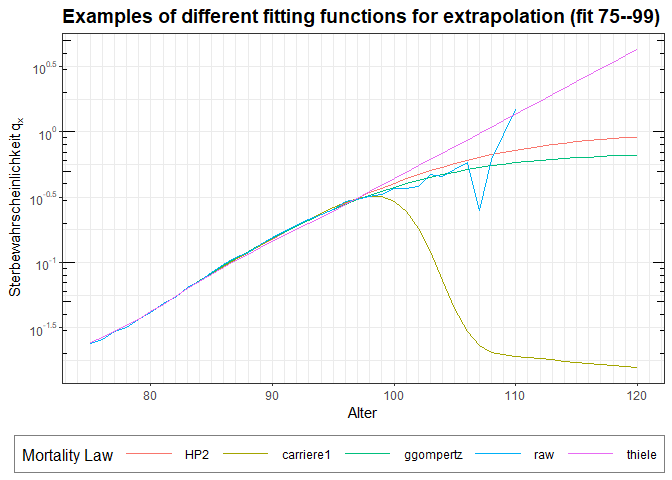The Austrian population mortality table for the year 2017 derived above is a period life table describing the observed mortality only in the year 2017. To describe death probabilities for a given person, one needs to take into account the mortality improvements and project the mortality into the future from the observation year. This can be done with age-dependent yearly mortality improvements, also called mortaltity trend $$\lambda_x$$.

For simplicity, we will use the trend $$\lambda_x$$ of the medium scenario of the mortality forecast of the Statistik Austria (forecast from 2016 to roughly 2080). These forecast tables are available as the mortality table mort.AT.forecast for male and female separately. Even though we derived a table for unisex, we will apply the male trends for simplicity. In practice, of course you would derive proper unisex trends from the available data.

mortalityTables.load("Austria_PopulationForecast")
plotMortalityTrend(mort.AT.forecast, title = "Forecast trend (medium scenario) by Statistik Austria")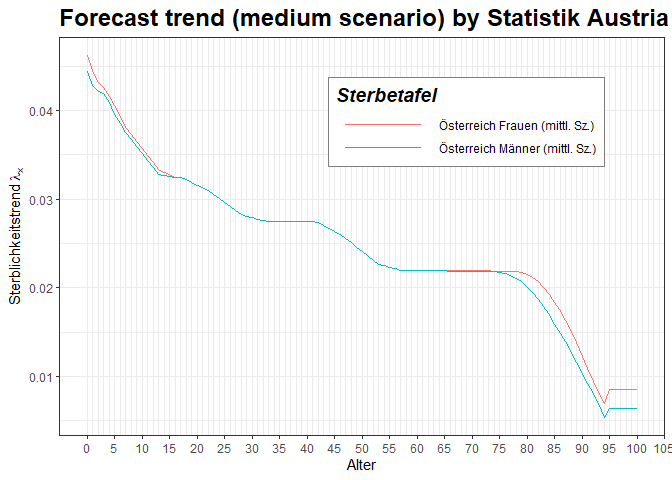As we can see, the trends appear to be derived from data until age 94 and then set to a constant value (“floor”). Let us first apply the male trend to the observed period life table of the year 2017, and then extrapolate the trend from age 94 to higher ages by an exponential function towards zero. The first can be done with the function mT.addTrend(), while the second can be done with mT.extrapolateTrendExp():

PopulationTable.AT2017.trend = PopulationTable.AT2017.ex %>%
mT.addTrend(mort.AT.forecast$m@trend, trendages = ages(mort.AT.forecast$m)) %>%
mT.setDimInfo(type = "smoothed, extrapolated, trend")

PopulationTable.AT2017.trend.ex = PopulationTable.AT2017.trend %>%
mT.extrapolateTrendExp(95) %>%
mT.setDimInfo(type = "smoothed, extrapolated, trend extrapolated")

plotMortalityTrend(PopulationTable.AT2017.trend, PopulationTable.AT2017.trend.ex,
title = "Extrapolating the trend via Exponential function") +
aes(color = type)
#> Warning: Removed 20 rows containing missing values (geom_line()).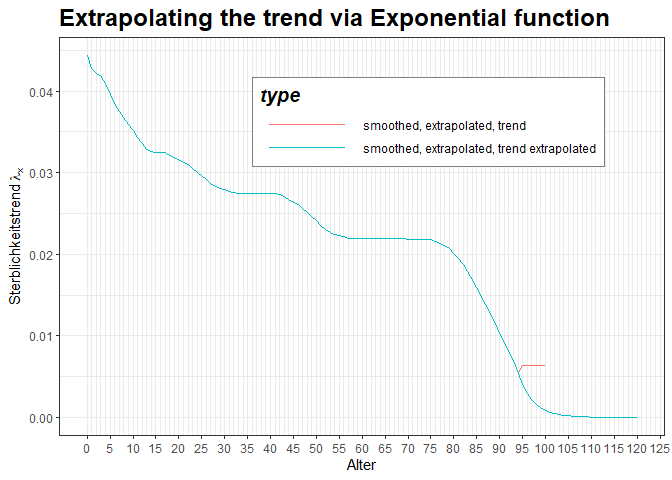plotMortalityTables(PopulationTable.AT2017, PopulationTable.AT2017.smooth, PopulationTable.AT2017.ex, PopulationTable.AT2017.trend.ex, YOB = 1980, title = "Austrian population mortality (Period 2017 vs. Generation 1980)", legend.position = c(0.01, 0.99), legend.justification = c(0,1))  +
aes(colour = type)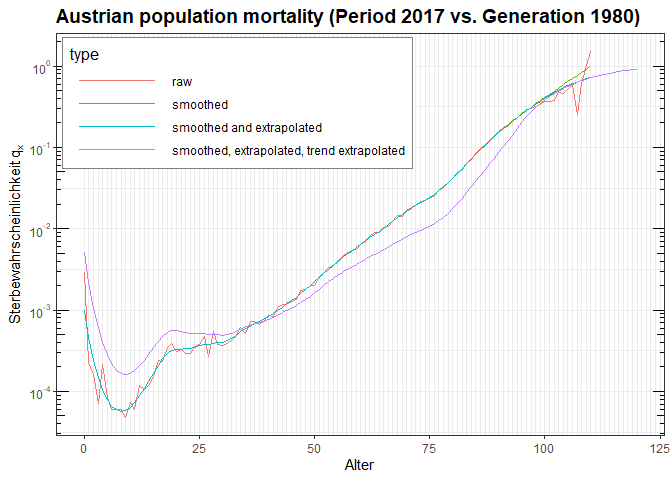So we have now started from raw data, calculated the death probabilities, smoothed them using Whittaker-Henderson, extrapolated to very old ages and added a trend to create a nice Cohort Life Table. We could now store the PopulationTable.AT2017.trend.ex in an .RData file and distribute it to the public. However, we might miss that all our modification were also recorded inside the mortality table (to allow later introspection into what was done and what was the result). For a published table, this might not be desired, so we first need to clean this additional support data with the mT.cleanup() function, which does not modify the table itself, but only removes all non-essential supporting information from the table:

# Lots of non-essential or support information is stored inside the table's data field:
PopulationTable.AT2017.trend.ex@data$whittaker #>$weights
#>    9.902640e-03 9.906213e-03 9.790593e-03 9.633836e-03 9.526789e-03
#>    9.507835e-03 9.578069e-03 9.441193e-03 9.378177e-03 9.433871e-03
#>   9.457978e-03 9.565714e-03 9.682226e-03 9.623760e-03 9.729914e-03
#>   9.716248e-03 9.834353e-03 1.011500e-02 1.041901e-02 1.099756e-02
#>   1.181051e-02 1.201924e-02 1.235036e-02 1.289431e-02 1.336379e-02
#>   1.359387e-02 1.372999e-02 1.362733e-02 1.371012e-02 1.366066e-02
#>   1.345438e-02 1.351974e-02 1.364485e-02 1.359768e-02 1.379285e-02
#>   1.393752e-02 1.375612e-02 1.301095e-02 1.271099e-02 1.250115e-02
#>   1.247615e-02 1.276387e-02 1.328318e-02 1.331546e-02 1.369437e-02
#>   1.431341e-02 1.474252e-02 1.535602e-02 1.610024e-02 1.627307e-02
#>   1.624561e-02 1.614926e-02 1.640552e-02 1.622923e-02 1.614036e-02
#>   1.559669e-02 1.497483e-02 1.440294e-02 1.380555e-02 1.326929e-02
#>   1.297614e-02 1.235557e-02 1.150436e-02 1.096556e-02 1.067262e-02
#>   1.024972e-02 1.007565e-02 1.007801e-02 1.041158e-02 1.033101e-02
#>   9.974142e-03 7.170591e-03 8.164682e-03 8.929619e-03 8.506759e-03
#>   9.195433e-03 9.656996e-03 1.012315e-02 7.949747e-03 5.845014e-03
#>   5.360872e-03 5.043744e-03 4.724615e-03 4.477298e-03 4.308243e-03
#>   3.996696e-03 3.728356e-03 3.335648e-03 2.879870e-03 2.421901e-03
#>   2.071341e-03 1.775088e-03 1.462955e-03 1.164619e-03 9.120986e-04
#>   6.678433e-04 4.593908e-04 2.819566e-04 1.294273e-04 6.713604e-05
#>  4.608583e-05 2.945073e-05 2.359083e-05 1.503001e-05 8.048140e-06
#>  4.288931e-06 1.918822e-06 7.934465e-07 3.830824e-07 0.000000e+00
#>  1.932463e-08

# Clean up the table (remove all non-essential data, but do not modify results)
PopulationTable.AT2017.Cohort.FINAL = PopulationTable.AT2017.trend.ex %>%
mT.cleanup() %>%
mT.round(digits = 6) %>%
mT.setName("Austrian Population Mortality, Period 2017 with trend projection")

Other functions that might be useful before publishing a table are: * mT.translate(), which simply moves the base year of the internal representation of a cohort life table to a different year (by applying the trend according to the translation), but leaves cohort death probabilities unchanged. * mT.round(), which rounds the probabilities of the base table and the trend to the given number of digits.

When using a population mortality table like the one we just derived in insurance contracts, the actuary often considers adding a certain security loading (e.g. 25% on all death probabilities) to ensure sufficient security and ensure the legal requirement of a prudent person. This can be done with the function mT.scaleProbs():

TableForProduct = PopulationTable.AT2017.Cohort.FINAL %>%
mT.scaleProbs(factor = 1.25, name.postfix = "10% security added")

plotMortalityTables(TableForProduct, PopulationTable.AT2017.Cohort.FINAL,
title = "Adding a security loading of 25%", Period = 2017, legend.position = "bottom")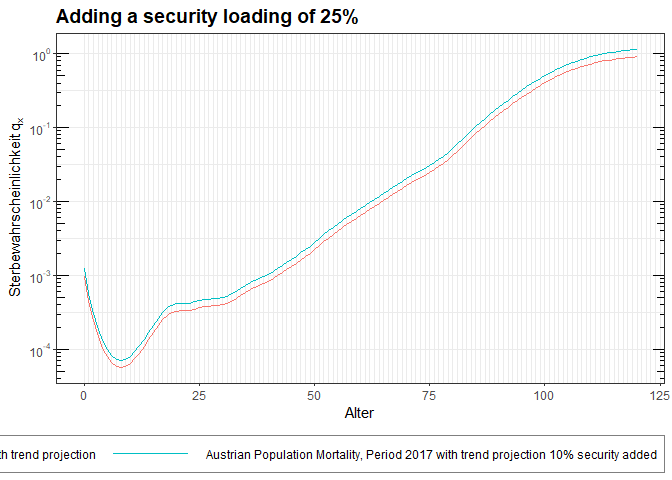# 8 Pension Tables

Pension tables generalize mortality tables in that the state space is increased from two states (alive / dead) to four states (active / invalidity or realy retirement / old age retirement / dead). As a consequence, there is no longer just one transition probability, but multiple.

Possible states are:

• active: healty, no pension, typically paying some kind of premium
• incapacity: disablity pension (in most cases permanent), not working, early pension
• retirement: old age pension, usually starting with a fixed age
• Widow/widower pension (if a widow exists at the time of death)

Correspondingly, the pensionTable class offers the following slots describing transition probabilities for the corresponding state transitions (by a mortalityTable-derived object):

• qx
death probability of actives (active -> dead)}
• ix
invalidity probability (active -> incapacity)}
• qix
death probability of invalid (invalid -> dead)}
• rx
reactivation probability (incapacity -> active)}
• apx
retirement probability (active -> retirement), typically 1 for a fixed age}
• qpx
death probability of retired (retired -> dead)}
• hx
probability of a widow at moment of death (dead -> widow), y(x) age differene}
• qxw
death probability of widows/widowers}
• yx
age difference of widow(er) at moment of death}
• qgx
death probability of total group (irrespective of state)}

All functions that handle mortalityTable object can be used with these slots.

Additionally, the following functions are provided to obtain the set of all transition probabilities in one data frame:

• transitionProbabilities(pension_table, YOB)
• periodTransitionProbabilities(pension_table, Period)